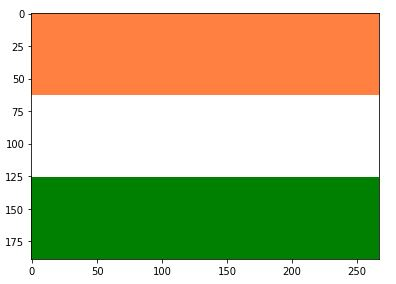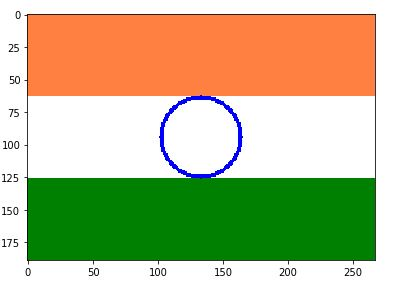Related Articles
Program to make Indian Flag in Python | Step by Step
• Difficulty Level : Medium
• Last Updated : 07 Nov, 2018

Here, We’ll be making Indian Flag in Python using Spyder tool which is a part of Anaconda interface. It is the beginner’s practice problem for python programmers.

Prerequisite: The only prerequisite for this code is little knowledge of basic python programming. You may install anaconda from here if you don’t have any interpreter for python.

Approach: We will be using simple characteristic equations of lines and circles in this code. It is recommended to try on your own before getting to the solution altogether.

Import these 3 packages for the functions used in this code.

```import skimage               # for using image processing functions
import os
from skimage import io       # for using imshow function
```

1. Read an image of at least the expected dimensions of the flag.

```image2 = io.imread('C:\Users\geeksforgeeks\Desktop\index.jpg')
```

2. Let us assume the dimensions of the flag to be 189px high and 267px wide.

```x = 189/2
y = 267/2
r = 189/6
```

3. Start two for loops to iterate to every pixel of the image.

```for i in range(0, 189):
for j in range(0, 267):
```

Inside the inner loop perform 3-(a) and 3-(b).

3-(a). By dividing the image into 3 equal part height-wise color the image in orange, white and green.

```if i in range(0, 189/3):
image2[i][j] = [255, 128, 64]       # Color coding for Orange
elif i in range(189/3, 189*2/3):
image2[i][j] = [255, 255, 255]      # Color coding for White
else:
image2[i][j] = [0, 128, 0]          # Color coding for Green
```io.imshow(image2)

3-(b). Now it’s time to draw the circle at the center for the Ashoka Chakra, using equation of circle.

```if (( pow((x-i), 2) + pow((y-j), 2) ) = pow(r-2, 2)):
image2[i][j] = [0, 0, 255]
```io.imshow(image2)

4. To draw spokes of the Chakra, we need to use equation of lines which stays inside the circle only, here I have drawn 4 x 2 spokes, however all 24 spokes can be drawn by using 12 equations as each line leads to two opposite spokes.

```for i in range(189/3, 189*2/3):
for j in range(267/3, 267*2/3):
if ((i == 189/2) or (j == 267/2) or (i+39 == j or
(i+39+j == 266))) and (( pow((x-i), 2) +
pow((y-j), 2) ) <= pow(r, 2)) :
image2[i][j] = [0, 0, 255]

```

5. It’s time to view our image now so we will use <imshow function.

```io.imshow(image2)
```

And Over Indian Flag in just 5 steps.

The overall code will look something like this…

 `"""``@author: geeksforgeeks``"""``# Packages``import` `skimage``import` `os``from` `skimage ``import` `io`` ` `# Reading image file``image2 ``=` `io.imread(``'C:\Users\KRISHNA\Desktop\index.jpg'``)`` ` `# Setting dimensions boundaries``x ``=` `189` `/` `2``y ``=` `267` `/` `2``r ``=` `189` `/` `6`` ` `# Iterating to all the pixels in the set boundaries``for` `i ``in` `range``(``0``, ``189``):``    ``for` `j ``in` `range``(``0``, ``267``):``        ``if` `i ``in` `range``(``0``, ``189` `/` `3``):``            ``image2[i][j] ``=` `[``255``, ``128``, ``64``]     ``# Orange Color``        ``elif` `i ``in` `range``(``189` `/` `3``, ``189` `*` `2` `/` `3``):``            ``image2[i][j] ``=` `[``255``, ``255``, ``255``]    ``# White Color``        ``else``:``            ``image2[i][j] ``=` `[``0``, ``128``, ``0``]        ``# Green Color``             ` `            ``# Equation for 2px thick ring``        ``if` `(( ``pow``((x``-``i), ``2``) ``+` `pow``((y``-``j), ``2``) ) <``=` `pow``(r ``+` `1``, ``2``)) ``and``(( ``pow``((x``-``i), ``2``) ``+` `pow``((y``-``j), ``2``) ) >``=` `pow``(r``-``2``, ``2``)):``            ``image2[i][j] ``=` `[``0``, ``0``, ``255``]        ``# Blue Color``             ` `# Iterating within the ring ``for` `i ``in` `range``(``189` `/` `3``, ``189` `*` `2` `/` `3``):``    ``for` `j ``in` `range``(``267` `/` `3``, ``267` `*` `2` `/` `3``):``         ` `        ``# Equation to draw 4 straight lines``        ``if` `((i ``=``=` `189` `/` `2``) ``or` `(j ``=``=` `267` `/` `2``) ``or` `(i ``+` `39` `=``=` `j ``or` `(i ``+` `39` `+` `j ``=``=` `266``))) ``and` `(( ``pow``((x``-``i), ``2``) ``+` `pow``((y``-``j), ``2``) ) <``=` `pow``(r, ``2``)) :``            ``image2[i][j] ``=` `[``0``, ``0``, ``255``]        ``# Blue Color`` ` `io.imshow(image2)                           ``# Output`

Output:Output

Attention reader! Don’t stop learning now. Get hold of all the important DSA concepts with the DSA Self Paced Course at a student-friendly price and become industry ready.  Get hold of all the important mathematical concepts for competitive programming with the Essential Maths for CP Course at a student-friendly price.

In case you wish to attend live classes with industry experts, please refer Geeks Classes Live and Geeks Classes Live USA

My Personal Notes arrow_drop_up